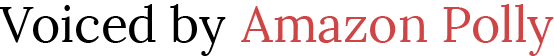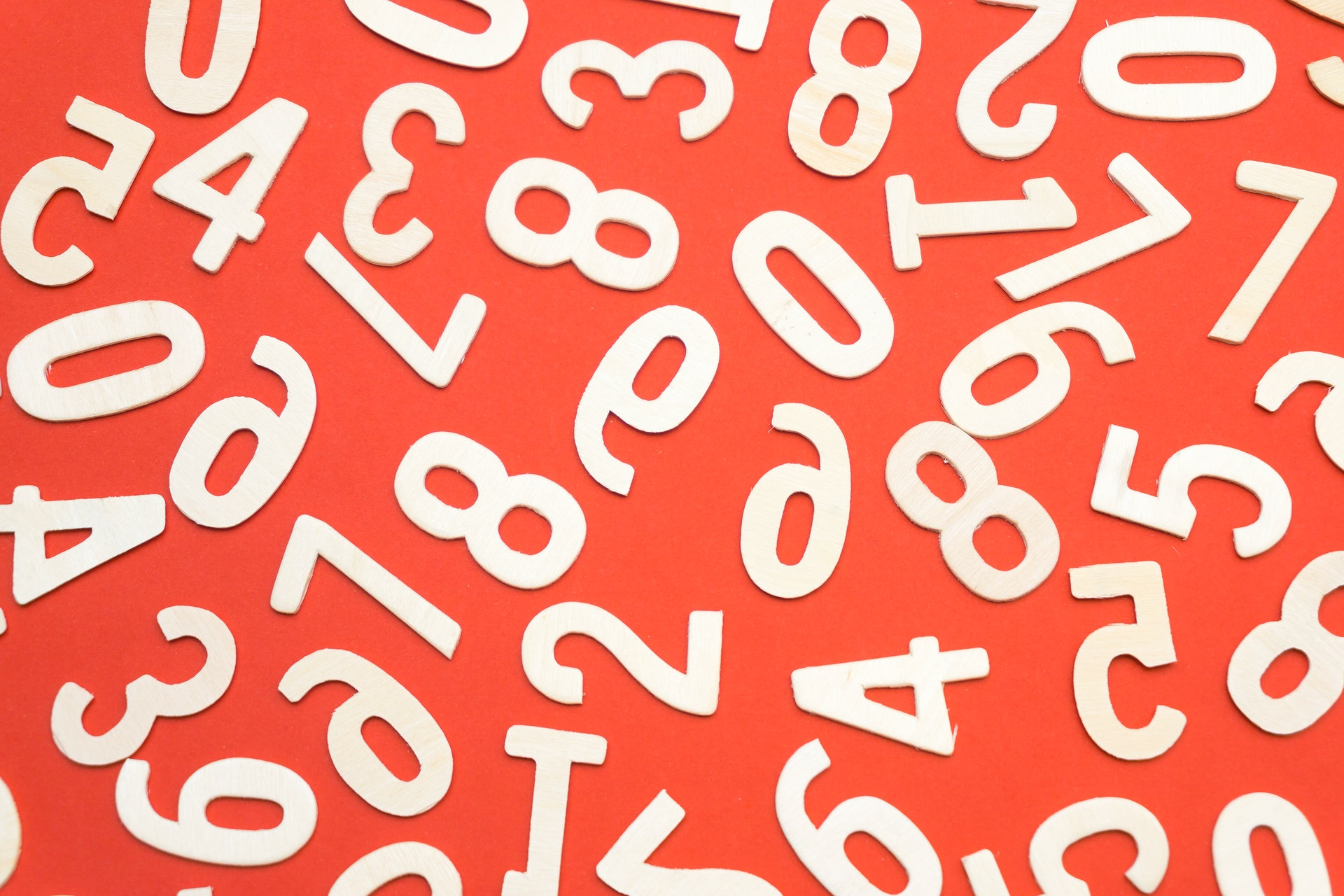# Teaching Operations on Integers

 Would You Rather Listen to the Lesson?When learning algebra, students learn how to perform different operations on integers, such as adding or subtracting integers, as well as multiplying and dividing them, by applying the appropriate rules for each operation.

Teaching children integer operations can sometimes be challenging and children can easily mix up all those plusses and minuses! To help out, we’ve compiled a list of teaching tips that will prepare children to perform integer operations with ease!## Operations on Integers Definition

Remind children what integers are – you can define them as whole numbers that also include negative numbers. So, integers can be positive or negative, and just like all whole numbers, they can’t be fractions or decimals. Provide a few examples on the whiteboard, such as:

– 7, + 43, 789, – 13, – 4, 340…

You may also want to review our article on teaching integers and absolute value for additional information.

Then, you can explain that when we talk about ‘operations on integers’, we’re referring to operations involving positive and negative integers, including addition, subtraction, multiplication, and division.

Explain that adding integers follows a few simple rules. If the signs of both numbers are the same, then we keep their signs and add the numbers. Provide examples of this, including two positive numbers and two negative numbers.

(+ 5) + (+ 7) = 12 (both numbers are positive, so we keep the ‘+’ sign in the sum)

(- 5) + (- 7) = – 12 (both numbers are negative, so we keep the ‘-’ sign in the sum)

Make sure to also point out that (- 5) + (- 7) is the same as – 5 – 7, and (+ 5) + (+ 7) is simply 5 + 7.

Then, explain what happens when we’re adding numbers with different signs. In this case, the smaller number is subtracted from the larger one. Illustrate this with a few examples, such as:

– 7 + 5 = – 2

+ 13 + (- 20) = 7

### Subtracting Integers

Explain that when subtracting integers, we keep the sign of the first number. Then, we change the subtraction operation to addition and change the sign of the next numbers to their opposites. In other words, positive numbers become negatives, and negative ones become positive.

This means that subtracting an integer is simply adding their opposite. Illustrate this with a few examples, such as:

(- 3) – (+ 2) = (- 3) + (- 2) = – 5

(- 11) – (- 2) = (- 11) + (+ 2) = – 9

### Multiplying Integers

Point out that when multiplying integers with the same signs, the product is always positive. When multiplying integers with different signs, the product is always negative. This makes integer multiplication much simpler than subtraction or addition. Provide a few examples:

(- 5) x (- 2) = 10

3 x 5 = 15

(- 2) x (+10) = – 20

– 7 x 4 = – 28

Make sure to also add that when multiplying more than two integers, we simply multiply one integer at a time to avoid mistakes. For instance:

4 x (- 2) x 8 = (- 8) x 8 = – 64

### Dividing Integers

Finally, explain that integer division simply follows the same rules as integer multiplication. That is, when dividing integers with the same sign, the quotient is always positive, whereas when dividing integers with different signs, the quotient is always negative.

Present a few examples, such as:

(- 25) (- 5) = 5

10 5 = 2

(- 8) 2 = – 4

## Integer Operations Activities

Use the following activities to practice operations with integers with your students. Most activities can easily be adjusted for individual exercises, so homeschooling parents can also benefit from them.

### Jack and Jill Integer RiddleThis awesome activity will help children review their knowledge of different integer operations. To play the Jack and Jill Integer Riddle, simply print out page 16 of this Interactive Notebook Worksheet (Members Only).

Divide students into groups of 3, 4 and distribute copies of the worksheet in each group. Explain to students that the game contains a riddle about Jack and Jill:

“Jack and Jill are lying on the floor inside the house, dead. They died from lack of water. There is shattered glass next to them. How did they die?”

Tell students that they’re supposed to solve the riddle as quickly as they can by performing the indicated integer operation that the riddle contains. They should match each letter to the blanks over the numbers on page 16 of this worksheet (Members Only) and thus get the answer to the riddle.

The first group to solve the riddle wins the game!

### Integers Jeopardy Game

This is a great online game that has a multi-player option. Divide students into groups of 3. Each student picks their avatar for the game and answers a math question related to either adding, subtracting, or multiplying integers.

Player 1 scores x points if they answer correctly (the number of points scored varies based on the difficulty of the problem) and then they let player 2 answer a math question. Player 2 repeats the same procedure. In the end, the person with the most points wins the game!

### Orbit IntegersIn this fun online game, players are supposed to engage in an intergalactic space race. Each player is shown a math problem related to adding positive and negative integers that they’re supposed to solve as quickly as they can.

By solving a problem correctly, they fuel the intergalactic space racer with math power – that’s why speed is crucial in this orbit game! In the end, each player gets a report of both their accuracy and their speed, so the game can also be played as a competition between two students.

## Before You Leave…

If you liked our tips in this article, make sure to check out our lesson and worksheets on operations on integers in our MathTeacherCoach community or head over to our blog. Sign up for our emails to receive loads of free content for children of all ages!